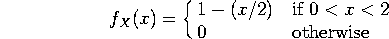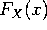M362K Final Exam
May 12, 2001

Problem 1. Cheating

In large, lower-division classes, 90% of professors apply a ``watch them like a hawk'' policy to prevent cheating, while 10% rely on an honor code. Cheating on exams is a serious problem in 20% of the classes with an honor code, and in 30% of the classes without an honor code.

a) What is the probability of a randomly chosen class having a Serious Cheating Problem?

b) If a class does have a Serious Cheating Problem, what is the probability that the class is being run on an honor code?

[Note: the numbers in this problem are made up, and reflect my own perception of reality. I strongly believe in honor codes, but I'm not so naive as to believe that everybody is honest. Rather, I believe that a) honest students have a right to be treated with dignity and trust, and b) the best way to make somebody trustworthy is to trust him.]

Problem 2. Big Six

In the game of Big Six, a player rolls four dice. If three or more of the dice show a 6, the player wins.

a) Compute the probability of winning.

b) A person plays the game 216 times. Let X be the number of times he wins. What type of random variable is X?

c) Find the expectation of X.

Problem 3. Manipulating pdfs

A continuous random variable X has pdf:a) Compute the cdffor all values of x (not just between 0 and 2.)

b) Compute the probability that X < 1/2.

c) Find the mean and variance of X.

Problem 4. A knotty problem

The number of knots in a piece of lumber is described by a Poisson distribution, with an average of 0.08 knots per square foot.

a) What is the probability that a 10 square foot piece of lumber has zero knots in it?

b) I buy a 10 square foot piece and a 15 square foot piece. What is the probability that I wind up with exactly 3 knots. (Simplify as much as possible).

c) If I purchase 1250 square feet of lumber, estimate the probability of my getting 85 or fewer knots.

Problem 5. Summer heat

The high temperature for a day can be described by a normal distribution with a certain mean and standard deviation. For July 10, the average high is 94.5 degress, and the standard deviation is 6.0 degrees. For July 23, the average high is 95.4 degrees and the standard deviation is 8.0 degrees.

a) What is the probability that the high on July 10 will be under 90.1 degrees.

b) What is the probability that the high on July 23 will be over 100.0 degress.

c) What is the probability that the high on July 23 will be hotter than the high on July 10? You may assume that the temperature on July 23 is independent of the temperature on July 10.

Problem 6. The Senate

The United States Senate has 100 members, two from each state. Currently, 87 are men and 13 are women. A committee of 50 is chosen at random from among the 100 US Senators.

a) What is the probability that the committee will have 43 men and 7 women?

b) What is the probability that each state will be represented on the committee? (Note: Each state has exactly two senators).

Problem 7. Sample Spaces

Two positive numbers, X and Y, are picked so that X is less than Y, and so that the sum of X and Y is less than 4.

a) Draw a picture of the sample space S.

b) Draw a picture of the event ``Y < 3''.

c) Suppose the joint probability is uniform (that is, the probability of a subset of S is proportional to its area). Compute the probability that Y < 3.